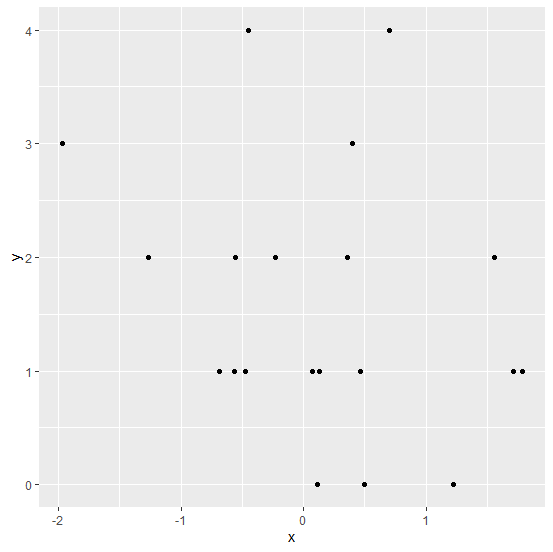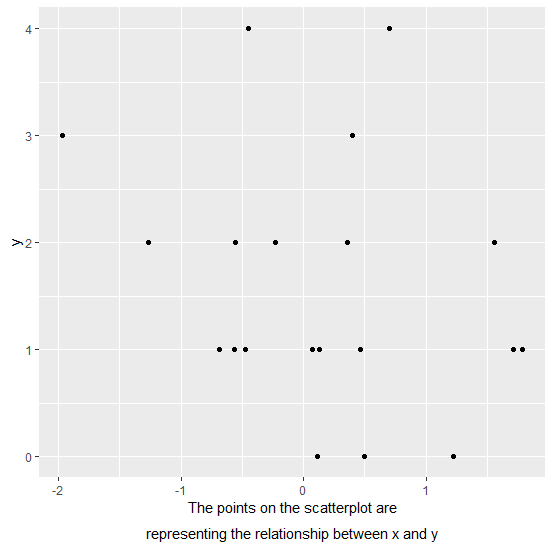# How to write a long line for the X-label of a scatterplot in R using ggplot2?

When we create a plot in R, the variable names are automatically plotted as axes labels but sometimes we want to give a brief detail of the X-label or a Y-label. If that brief is not small so that the expression function can contain the length of the label then it becomes difficult but it can be done with the help of atop inside expression.

## Example

Consider the below data frame −

Live Demo

set.seed(123)
x<-rnorm(20)
y<-rpois(20,2)
df<-data.frame(x,y)
df

## Output

    x    y
1 -0.56047565 1
2 -0.23017749 2
3 1.55870831 2
4 0.07050839 1
5 0.12928774 1
6 1.71506499 1
7 0.46091621 1
8 -1.26506123 2
9 -0.68685285 1
10 -0.44566197 4
11 1.22408180 0
12 0.35981383 2
13 0.40077145 3
14 0.11068272 0
15 -0.55584113 2
16 1.78691314 1
17 0.49785048 0
18 -1.96661716 3
19 0.70135590 4
20 -0.47279141 1
library(ggplot2)
ggplot(df,aes(x,y))+geom_point()

## OutputCreating the plot with lengthy X-label −

ggplot(df,aes(x,y))+geom_point()+
+ xlab(expression(atop("The points on the scatterplot are",paste("representing the relationship between x and y"))))

## Output